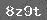# 小学数学第三册第四单元试卷

1.2. 先圈一圈，再写出除法算式．□÷□=

8里面有□个2

3. (1)10除以5等于2．□○□=

(2)被除数是12，除数是6，商是2．□÷□=

4. 12个△，每6个一份，能分成几份？

12÷□=

5. (1)15÷3=5表示把(　　　 )平均分成(　　　 )份，每份是(　　　 )

(2)12÷4=3表示把(　　　 )平均分成(　　　 )份，每份是(　　　 )

6. 12个○，平均分成4份，每份是几个？

12÷□=

7. (1)10÷2=5　 表示把(　　　 )个东西，每(　　　 )个一份，分成了(　　　 )份．

(2)20÷4=5　 表示把(　　　 )个东西，每(　　　 )个一份，分成了(　　　 )份．

8.(1)20平均分成4份，每份是5．□÷□=

(2)15平均分成5份，每份是3．□÷□=

9.(1)8÷2=4 表示把(　　　 )个东西，每(　　　 )个一份，分成了(　　　 )份．

?(2)8÷4=2 表示把(　　　 )个东西，每(　　　 )个一份，分成了(　　　 )份．

10. (1)8÷4=2表示把(　　　 )平均分成(　　　 )份，每份是(　　　 )

(2)18÷3=6表示把(　　　 )平均分成(　　　 )份，每份是(　　　 )

11. 12个图片，平均分成3份，每份是几个？

12÷□=

12. 12个△，每21份，能分成几份？

12÷□=

13.1.15÷3(　　　 )

2.10÷5(　　　 )

14. 写出除法算式．

(1)6里面有(　　　 )2．□÷□=

(2)8里面有(　　　 )4．□○□=

1.5×3=　　　4×6=　　　6×6=

14-7=　　　2×2=　　　4×4=

3×3=　　　86-4=　　　28+9=

2.　4×3=　　　　　 2+3=　 　　　　　1×5=

5×4=　　　　　 3-2=　　　　　　 2×6=

6×1=　　　　　2×3=　　　　　 　4×2=

### 相关推荐

 留言与评论（共有 0 条评论）

 验证码：